# Motion Class 9 Important Questions Science Chapter 8

## Motion Class 9 Important Questions and Answers Science Chapter 8

Based on Motion of an object along a straight line

Question 1.
What do you understand by the motion of an object ?
An object is said to be in motion if its position change:, with time.

More Resources

Question 2.
What is a reference point ?
A fixed point with respect to which the given object changes its position.

Question 3.
Is it possible that the train in which you are sitting appears to move while it is at rest ?
Yes. The train at rest in which we are sitting appears to move when we look at another train moving parallel to the track on which our train is standing.

Question 4.
Define the term “distance”. –
The length of actual path travelled by an object in the given time is called the distance travelled by the object.

Question 5.
Is distance a scalar or a vector quantity ?
Distance is a scalar quantity’.

Question 6.
Define the term “displacement”.
The shortest distance between the initial and final positions of the object in a particular direction.

Question 7.
Is displacement of an object a scalar or a vector quantity ?
Displacement is a vector quantity.

Question 8.
Under what condition is distance and the magnitude of the displacement equal ?
(CBSE 2010, 2013, 2014, 2015)
Distance and magnitude of the displacement are equal if an object moves along a straight line in one direction.

Question 9.
A particle is moving in a circle of diameter 5 m. What is its displacement when it completes 1 ~ revolutions ?
After 1 revolution, particle is diametrically opposite to its initial position. Therefore, its displacement = diameter of the circle = 5 m.

Question 10.
What do you mean by uniform motion ? (CBSE 2010, 2012)
The motion of an object is uniform motion if it travels equal distances in equal intervals of time along a straight line.

Question 11.
What is non-uniform motion ? (CBSE 2012)
The motion of an object is non-uniform if it travels unequal distances in equal intervals of time.

Question 12.
Give one example of non-uniform motion. (CBSE 2012)
A bus moving on a hilly road or on a zig-zag road.

Question 13.
What is the shape of the path of a body when it is in uniform motion ?
Straight line.

Based on Speed and Velocity

Question 14.
Define the term “speed”.
The distance travelled by an object per unit time is known as speed of the object.

Question 15.
State SI unit of speed.
metre/second (ms-1)

Question 16.
Is speed a scalar or a vector quantity ?
Speed is a scalar quantity.

Question 17.
Define uniform speed.
The speed of an object is said to be uniform speed if it travels equal distances in equal intervals of time.

Question 18.
Define average speed. (CBSE 2012)
Average speed is the ratio of the total distance travelled by an object to the total time taken by it.

Question 19.
What does speedometer of an automobile measure ?
Speedometer of a vehicle measures its instantaneous speed.

Question 20.
Define the term “velocity”.
Velocity is defined as the ratio of the displacement of an object to the time taken by it.

Question 21.
What do you understand by a uniform velocity ?

Or

When is a body said to have uniform velocity ? (CBSE 2011, 2012, 2013)
Velocity of an object is uniform if it has equal displacements in equal intervals of time.

Question 22.
What do you understand by a non-uniform velocity ?

Or

When is a body said to have non-uniform velocity ? (CBSE 2011)
Velocity of an object is non-uniform if it has unequal displacements in equal intervals of time.

Question 23.
Define average velocity.
Average velocity is the ratio of the total displacement of an object to the total time taken by it.

Question 24.
Is velocity a scalar or a vector quantity ?
Velocity is a vector quantity.

Question 25.
An object starts with initial velocity u and attains final velocity V. The velocity is changing at a uniform rate.
What is the formula for calculating average speed in this situation ? (CBSE 2011)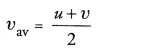Question 26.
A physical quantity measured is -10 m s-1. Is it a speed or velocity ?
It is velocity because velocity can be positive or negative. On the other hand, speed is always positive.

Question 27.
What is the numerical ratio of average velocity to average speed of an object when it is moving along a straight path ? (CBSE 2014)
In this case, both are equal, so ratio is 1.
Based on Acceleration

Question 28.
Define acceleration of a body.
Acceleration is defined as the change in velocity per unit time.

Question 29.
State SI unit of acceleration.

Question 30.
Is acceleration a scalar or a vector quantity ?
It is a vector quantity.

Question 31.
Why does (second)in the unit of acceleration ?
change in velocity change in displacement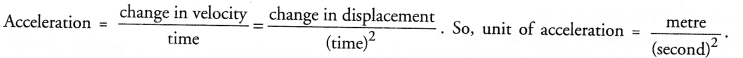Question 32.
Name the physical quantity that corresponds to the rate of change of velocity.
Acceleration.

Question 33.
What do you mean by positive acceleration ? (CBSE 2013, 2015)
When the change in velocity of a body takes place in the direction of motion of the body, then the acceleration is positive.

Question 34.
Give one example of positive acceleration.
The acceleration of a body is positive, when it falls from certain height.

Question 35.
What do you mean by negative acceleration ? (CBSE 2015)
When the change in velocity of a body takes place in a direction opposite to the direction of motion of the body, then the acceleration is negative.

Question 36.
Give one example of retardation or negative acceleration. (CBSE 2013)
When the velocity of a train decreases before entering the platform.

Question 37.
When do you say that a body is in uniform acceleration ? (CBSE 2010, 2013)

Or

Define uniform acceleration. (CBSE 2010, 2013, 2014, 2015)
When the velocity of a body changes by an equal amount in equal intervals of time, then the body is in uniform . acceleration.

Question 38.
When do you say that a body has non-uniform acceleration ? (CBSE 2013)
When the velocity of a body changes by unequal amount in equal intervals of time, then the body is in non¬uniform acceleration.

Question 39.
Give an example of a motion in which the acceleration is in the direction of motion.
When a body (say a ball) falls from the roof of a house, then the acceleration of the body is in the direction of motion.

Question 40.
Give an example of a motion in which the acceleration is against the direction of motion.
When a ball rolling over a horizontal surface slows down due to the force of friction between the ball and the horizontal surface, then acceleration is against the direction of motion.

Or

When a body is thrown upward, then the acceleration is against the direction of motion of the body.

Question 41.
What is the acceleration of a body moving with uniform velocity in a straight line ? (CBSE 2014)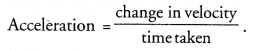Since velocity is uniform, so change in velocity = 0. Hence, acceleration of body = 0.
Based on distance-time and velocity-time graphs

Question 42.
Draw a distance-time graph for an object at rest.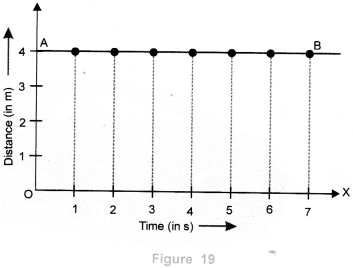Question 43.
What will you say about the motion of a body if its distance-time graph is a straight line having a constant angle with time axis ? (CBSE 2010)
If distance-time graph is a straight line having a constant angle with time axis, then the distance travelled by the body increases in equal amount in equal intervals of time. It means, the motion of the body is a uniform motion.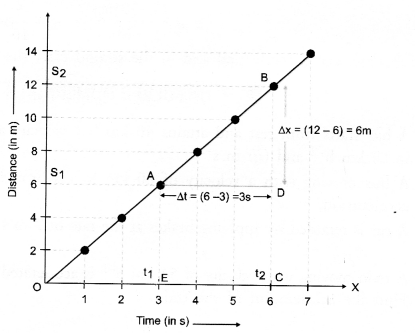Question 44.
If the displacement-time graph for a particle is parallel to time axis, how much is the velocity of the particle.
(CBSE 2013)
Velocity is zero as there is no change in displacement with time.

Question 45.
What does the slope of a velocity-time graph represent ?
(CBSE 2010, Term I)
It represents the acceleration of the body.

Question 46.
Figure shows the displacement-time graph of four children A, B, C and D. Which child has the highest velocity ? (CBSE 2010, 2012, 2013)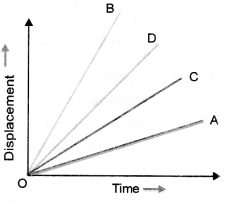Velocity = Slope of displacement – time graph.
Since slope of displacement-time graph for the child B is greater than all other children. Hence, child B has the highest velocity.

Question 47.
What can you say about the motion of a body if its velocity-time graph is a straight line parallel to time axis ?
(CBSE 2012)
When velocity-time graph of the motion of a body is a straight line parallel to time axis, then the velocity of the body does not change with time. So the body is said to move with constant velocity.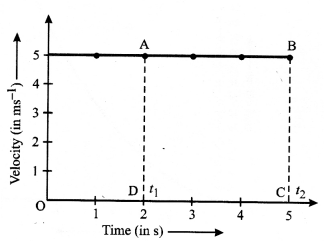Question 48.
Name the physical quantity measured by the area occupied below velocity-time graph for uniform motion.
(CBSE2012, 2014)
Magnitude of displacement of the body = area under v-t graph.

Question 49.
Plot velocity-time graph of a body moving with constant or uniform velocity. (CBSE 2012, 2014)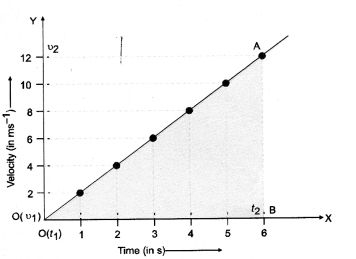Question 50.
Velocity-time graph of a body is shown in figure A.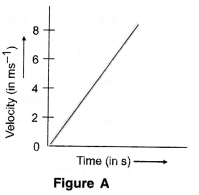What conclusion can be drawn about the type of motion ? (CBSE 2010, 2011, 2012)
Motion of the body is uniform motion.

Question 51.
Velocity-time graph of a body is shown in figure B.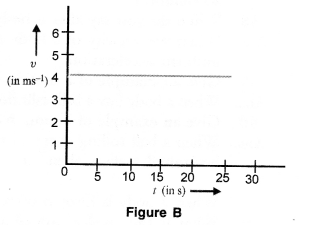What conclusion can be drawn about the velocity of the body from this graph ?
(CBSE Sample Paper 2010, 2011)
Body is moving with constant velocity = 4 m s-1.

Based on circular motion

Question 52.
Define circular motion. (CBSE 2011, 2012)
The motion of a body moving around a fixed point in a circular path.

Question 53.
State the meaning of uniform circular motion.
(CBSE 2010, 2012, 2013, 2014, 2015)
The circular motion of a body having constant speed is called uniform circular motion.

Question 54.
Give an example of a uniform circular motion. (CBSE 2014)
A cyclist travelling with constant speed on a circular path.

Question 55.
Is uniform circular motion accelerated motion ?
(CBSE 2012, 2013)
Uniform circular motion of a body is accelerated because its direction of motion changes continuously although its speed is constant.

Question 56.
If the acceleration of the particle is constant in magnitude but not in direction, what type of path does the particle follow.
Circular path.

Question 57.
Why is the motion of an athlete moving along the circular path an accelerated motion ?
The direction of motion of the athlete is changing continuously, so his velocity is also changing continuously. Due to change in velocity along the circular path, his motion is accelerated motion.

Based on motion of an object along a straight line

Question 1.
Can a body exist in a state of absolute rest or of absolute motion ? Explain with example. (CBSE 2015)
A state of rest or state of motion is not absolute but it is a relative term. A body at rest in one frame of reference
may be in motion in other frame of reference. For example, a passenger sitting in a moving bus is at rest with respect to the bus or other co-passengers. However, the same passenger is in motion with respect to a person standing on a road side. Thus, rest and motion are relative terms.

Question 2.
Distinguish between distance and displacement. (CBSE 2010, 2012, 2013)

 Distance Displacement 1. Distance is the length of the actual path travelled by an object in a given interval of time. 1. Displacement is the shortest distance between the initial and final positions of a moving object in a particular direction. 2. Distance travelled by an object depends upon the shape of the path followed by the object in going from initial position to the final position. Distance travelled by an object in a given interval of time is always positive. Distance is a scalar quantity. 2. The displacement of an object between initial and final positions of the object does not depend upon shape of the path followed by it. Displacement of an object in a given interval of time may be positive, negative or zero. Displacement is a vector quantity.

Question 3.

Mention two differences between speed and velocity. (CBSE 2013, 2015)

 Speed Velocity 1. Distance travelled by an object per unit time is known as its speed. The distance travelled by an object in a particular direction (i.e. displacement) per unit time is known as its velocity. 2. Average speed of a moving object cannot be zero. Average velocity of a moving object can be zero. 3. Speed tells how fast an object moves. Velocity tells how fast an object moves and in which direction it moves. 4. Speed is a scalar quantity. Velocity is a vector quantity. 5. Speed of an object is always positive. Velocity of an object can be positive or negative.

Question 4.
Give one example each to differentiate between uniform acceleration and non-uniform acceleration.
(CBSE 2010, 2012)
Object has uniform acceleration if its velocity changes by an equal amount in equal intervals of time. For example, motion of an object falling freely from the top of a building.
On the other hand if velocity of an object changes by an unequal amount in equal intervals of time, then it has non-uniform acceleration. For example, the motion of a bus on a hilly track or on a circular track.

Question 5.
Differentiate acceleration from velocity.

 Acceleration Velocity 1.      Acceleration of an object is defined as the change in velocity of the object per unit time. 2.       SI unit of a acceleration is m s-2. 1.     Velocity of an object is defined as the change in displacement of the object per unit time. 2.      SI unit of velocity is m s-1.

Based on distance-time and velocity-time graph

Question 6.
What kind of motion of a body is represented by the graphs shown in figure C ?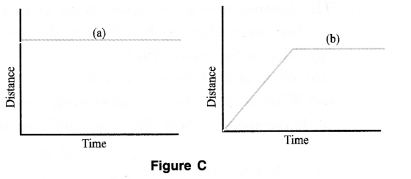(a) Body is at rest because its distance does not change with time.
(b) Body has uniform motion and then comes to rest.

Question 7.
Explain the differences between the two graphs shown in figure E.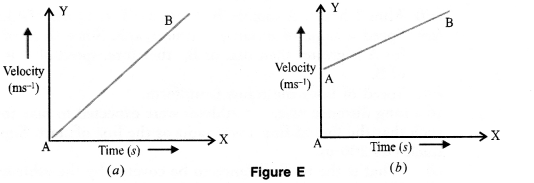Graph (a) shows that body starts from rest and then accelerates uniformly.
Graph (b) shows that body starts with some initial velocity (≠0) and then accelerates uniformly

Question 8.
Four speed-time graphs are shown in figure F :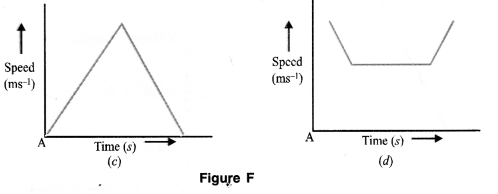Which graph represents the following cases ?
(a) A ball thrown vertically upwards and returning to the hand of the thrower.
(b) A body decelerating to a constant speed and then accelerating.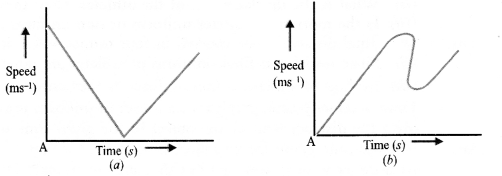(a) Graph (a)
(b) Graph (d).

Question 9.
What do the graphs (I and II) shown in figure G indicate ?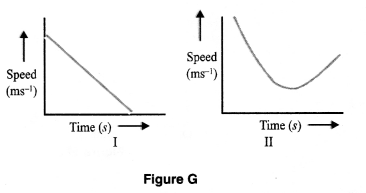Graph I indicates that a body has certain initial speed and then its speed decreases to zero. In other words, this graph shows the retarded motion of a body.
Graph II shows that a body first retards and then accelerates.

Question 10.
A person travelling in a bus noted the timings and the corresponding distances as indicated on the km stones.
(a) Name this type of table.
(b) What conclusion do you draw from this table ?
(a) The table gives distance-time data.

 Time Distance 8:00 am 10 km 8:15 am 20 km 8:30 am 30 km 8:45 am 40 km 9:00 am 50 km

(b) As the bus is travelling equal distances in equal intervals of time, so the motion of the bus is uniform motion.

Question 11.
The distance-time graph of trains are given in figure L.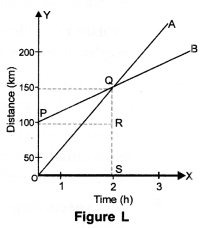The trains start simultaneously in the same direction.
(i) How much ahead of A is B when the motion starts ?
(ii) What is the speed of B ?
(iii) When and where will A catch B ?
(iv) What is the difference between the speeds of A and B ?
(v) Is the speed of both the trains uniform or non-uniform ? Justify your answer.
(i)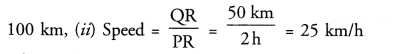(iii) After 2 hours, A catches B. A catches B at Q i.e. 150 km from origin O.
(iv) Speed = slope of distance – time graph. Since slope of distance – time graph for A is greater than that of B, therefore, speed of A is greater than the speed of B.
(v) Speed of both the trains is uniform.

Question 12.
In a long distance race, the athletes were expected to take four rounds of the track such that the line of finish was same as the line of start. Suppose the length of the track was 200 m.

1. What is the total distance to be covered by the athletes ?
2. What is the displacement of the athletes when they touch the finish line ?
3. Is the motion of athletes uniform or non-uniform ?

1. Total distance to be covered in four rounds = 4 x length of track = 4 x 200 = 800 m.
2. Since initial and final positions of athletes are same, so displacement of athletes = 0.
3. Motion of athletes is non-uniform as they cover unequal distances in equal intervals of time.

Question 13.
Draw a velocity-time graph for an object in uniform motion. Show that the area under the velocity-time graph gives the displacement of the object in the given time interval.
Velocity-time graph for an object in uniform motion is shown in figure M.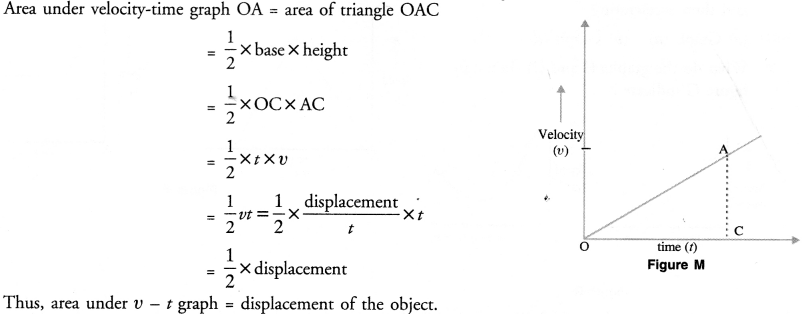Question 14.
Which of the two bodies A and B in the following graph is moving with higher speed and why ?
(CBSE 2015)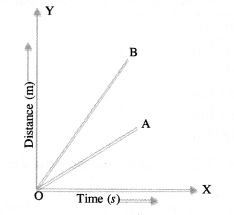Speed of a body = slope of distance-time graph.
Since slope of distance-time graph for body B is greater than the slope of distance-time graph for body A. Therefore, body B is moving with higher speed than body A.

Question 15.
Which of the two bodies A and B in the following graph is moving with a greater acceleration and why ?
(CBSE 2015)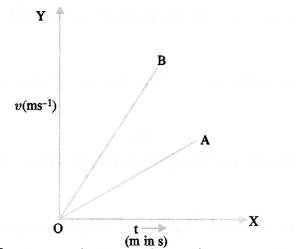Acceleration of a body = slope of V — t graph. Since slope of V — t graph for body B is greater than the slope of V – t graph for body A, therefore, body B is moving with a greater acceleration than the body A.

Based on equation of motion

Question 16.
State three equations of motion. Which of them describes :
(i) velocity-time relation
(ii) position-time relation ?
(CBSE 2013, 2015)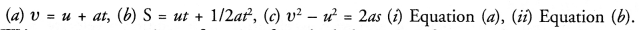Question 17.
Write any two equations of motion for a body having uniform acceleration.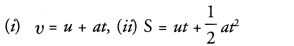Based on circular motion

Question 18.
What is uniform circular motion ? How is uniform circular motion regarded as an accelerated motion ? Explain. (CBSE 2010, 2011)
Circular motion of a body having constant speed is known as uniform circular motion.
When a body has uniform circular motion, its velocity changes due to the continuous change in the direction of its motion. Hence, the motion of the body is accelerated motion.

Question 19.
What is the difference between uniform motion in a straight line and uniform circular motion ?
(CBSE 2010, 2012, 2013)

 Uniform motion in a straight line Uniform motion in a straight line 1. The direction of motion of an object does not change. 1. direction of motion of an object changes continuously. 2. If an object moves with constant velocity, acceleration of the object is zero. 2. uniform circular motion, object moves with constant speed but its direction of motion changes continuously. Therefore, velocity of an object having uniform circular motion changes. Hence, the motion.

Question 20.
An object is moving with uniform speed in a circle of radius r. Calculate the distance and displacement
(a) When it completes half the circle,
(b) When it completes full circle,
(c) What type of motion does the object possess ?
(a) When object completes half the circle,
distance travelled by the object = ½ x circumference of the circle = ½ x 2πr
= πr. Displacement of the object = 2r  ( object is just at opposite position from its original position).
(b) Distance travelled by the object = circumference of the circle = 2πr. Displacement of the object = zero ( object’s initial and final positions are same).
(c) Since the direction of motion of the object changes continuously in the circle, hence its velocity changes and its motion is accelerated motion.

Question 1.
Draw velocity-time graph for an uniformly accelerated object. Using velocity-time graph, derive v = u + at.

Or

Derive the equation of motion V = u + at using graphical method. (CBSE 2011, 2013, 2015)
Derivation of First Equation of Motion From Velocity-Time Graph (Equation for Velocity-time Relation)
The velocity-time graph of a uniformly accelerated motion of the body having initial velocity (u) is shown in figure .
The velocity-time graph is a straight line AB.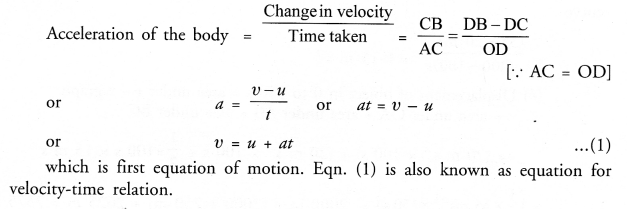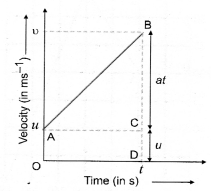Question 2.
Draw velocity-time graph for an uniformly accelerated object. Using velocity-time graph, derive S = ut + ½ at² .
(CBSE 2010, 2011, 2013, 2015)

Or

From velocity-time graph of a body, derive equation for “position-time” relation (II equation of motion) for a body. (CBSE 2012)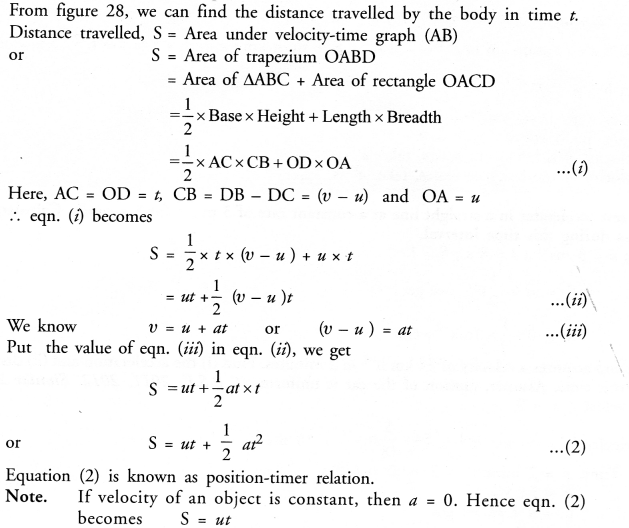Question 3.
Draw velocity-time graph for an uniformly accelerated object. Using velocity-time graph, derive v2 – u2 = 2aS.
(CBSE 2010, 2011, 2013)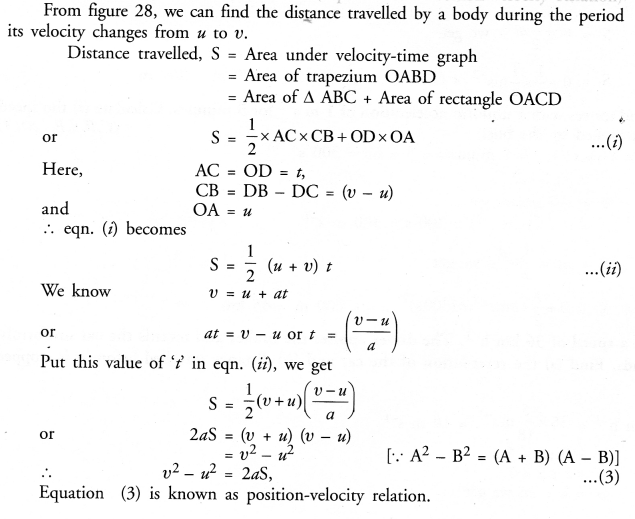Question 4.
Write the three equations for the uniformly accelerated motion. Also derive the second and third equations
by graphical method. (CBSE 2013)
Derivation of First Equation of Motion From Velocity-Time Graph (Equation for Velocity-time Relation)
The velocity-time graph of a uniformly accelerated motion of the body having initial velocity (u) is shown in figure 28.
The velocity-time graph is a straight line AB.Question 5.
An object starts a linear motion with velocity ‘u’ and with acceleration ‘a’ It acquires a velocity ‘v’ in time ‘t’.
(i) Draw its velocity-time graph
(ii) Obtain 1st equation of motion, v = u + at for velocity time relation using this graph. (CBSE 2013)